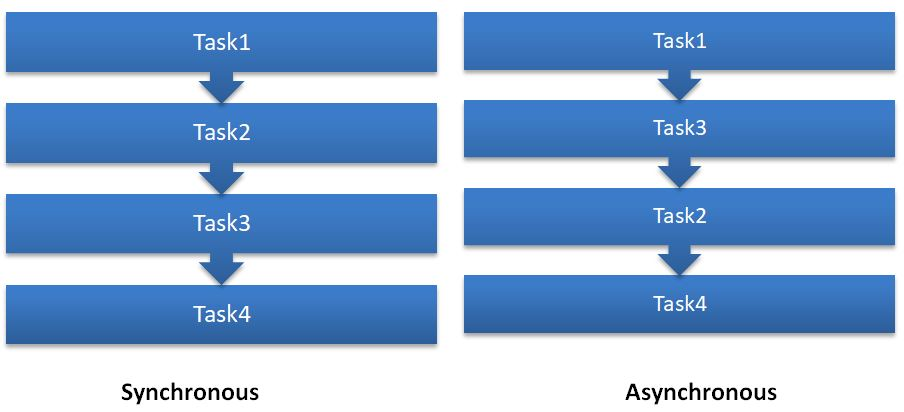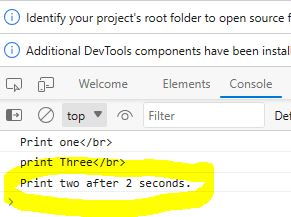﻿ JavaScript Synchronous and Asynchronous - TutorialsTrend
This Angular tutorial helps you get started with Angular quickly and effectively through many practical examples.
`

# JavaScript Synchronous and Asynchronous

In this tutorial, we'll discuss about JavaScript's synchronous and asynchronous programming.

JavaScript is a single-threaded language, but at the same time, can be made to be non-blocking, asynchronous, and concurrent. Take a look at the image below.#### Synchronous in JavaScript

In JavaScript, synchronous code is executed in a single thread, meaning that each operation is executed one after the other in a sequential way. This means that if one operation takes a long time to complete, it will block the execution of the subsequent operations until it's finished. So synchronous is a blocking operation.

JavaScript is an interpreted language, and the code runs line by line. So by default, JavaScript code execution is synchronous, which means the code runs in a particular sequence one by one only after the completion of the program.

Synchronous code can be useful for operations that don't require much processing time or when the order in which the operations are executed is important.

Example

In synchronous programming, each JavaScript statement or piece of code is run sequentially, and the programme waits for each operation to finish before going on to the next line.

See the following code example of synchronous JavaScript.

``````<!DOCTYPE html>
<html>
<body>
<h2>TutorialsTrend- JavaScript Synchronous Example</h2>
<script type="text/javascript">
function one() {
document.write("Callong one Function" +"</br>");

}
function two() {
document.write("Callong Two Function" +"</br>");
one();
}
function three() {
document.write("Callong Three Function" +"</br>");
two();
}
three();
</script>
</body>
</html>``````

In the above example, we have declared two functions, that is one() and two(), and now we are calling the two() function. The following output we will recieve.Here in ouput you can see output is in sequential.

#### Asynchronous in JavaScript

In JavaScript, asynchronous allows code to run concurrently, so there is no blocking of the execution flow. In asynchronous programming, the tasks are executed in the background while the other tasks continue to execute other statements, so basically, when a task gets completed, a callback function is invoked, and it allows the program to handle the result or to continue with the next statement.

Concurrently - other codes are allowed to run parallelly even without waiting for the current code to finish

JavaScript provides asynchronous programming mechanisms, such as callbacks, Promises, and async/await, which allow for non-blocking code execution and better performance.

Using asynchronous code can improve the performance and responsiveness of JavaScript applications, especially when dealing with long-running operations

Example

See the following code example of asynchronous JavaScript.

``````<!DOCTYPE html>
<html>
<body>
<h2>TutorialsTrend- JavaScript Asynchronous Example</h2>
<script type="text/javascript">
console.log("Print one");
setTimeout(() => console.log("Print two after 2 seconds."), 2000);
console.log("print Three");
</script>
</body>
</html>``````

OutputIn the above code example, if we see the output of the code, we notice that after printing "Print one", "Print three" is printed out in our console before "Print two after 2 seconds" and this is because of the asynchronous execution of the code here in the code example the setTimeout() function waits for 2 seconds.

#### Difference between Synchronous vs Asynchronous

 SNo. Synchronous Asynchronous 1 Synchronous programming Concurrent Execution 2 Blocking Nature Non-Blocking Nature 3 Simple Control Flow Event-driven Model

Prev Next